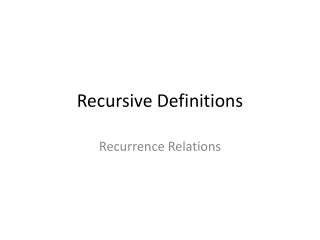DownloadDownload PresentationRecursive Definitions

# Recursive Definitions

Download Presentation## Recursive Definitions

- - - - - - - - - - - - - - - - - - - - - - - - - - - E N D - - - - - - - - - - - - - - - - - - - - - - - - - - -
##### Presentation Transcript

1. Recursive Definitions Recurrence Relations

2. Recursive Definitions • A recursive definition (or inductive definition) is used to define an object in terms of itself. • Recursive definitions have two parts: a base case (basis) and an inductive step.

3. Recursive vs circular defns • The difference between a circular definition and a recursive definition is that a recursive definition must always have base cases, cases that satisfy the definition without being defined in terms of the definition itself, and all other cases comprising the definition must be "smaller" (closer to those base cases that terminate the recursion) in some sense. • In contrast, a circular definition may have no base case, and define the value of a function in terms of that value itself, rather than on other values of the function.

4. Recurrence Relation • A recurrence relation is an equation that recursively defines a sequence: each term of the sequence is defined as a function of the preceding terms.

5. Recursively Defined Set • Recursively defined set: a set of objects on which no ordering is imposed (different from sequence).

6. BNF Exercise • Express the following in BNF: • A floating-point binary number consists of an optional sign followed by one or more bits, followed by the letter E, followed by another optional sign, followed by one or more bits. Examples: 11010E-1010, -100101E11101, and +1E0.

7. Recurrence Relations